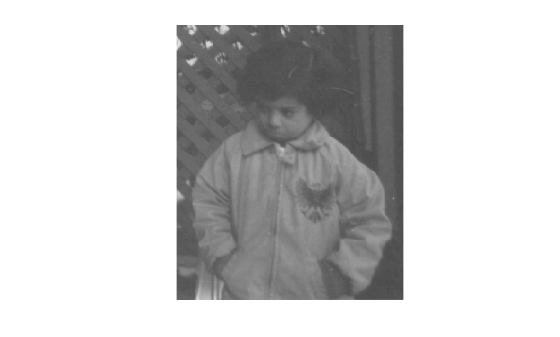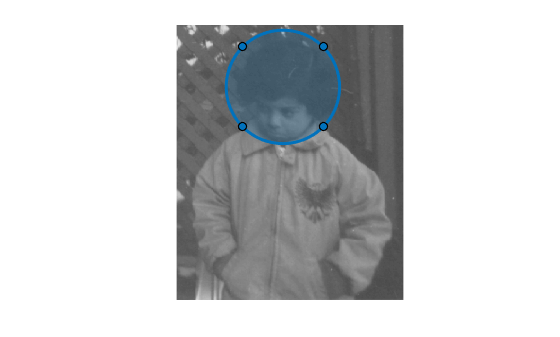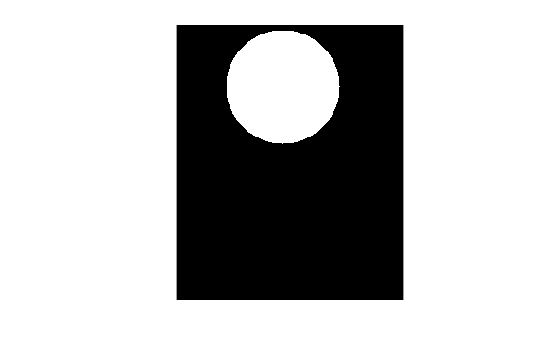Main Content

## Create a Binary Mask

Image Processing Toolbox™ supports four methods to generate a binary mask. The binary mask defines a region of interest (ROI) of the original image. Mask pixel values of `1` indicate the image pixel belongs to the ROI. Mask pixel values of `0` indicate the image pixel is part of the background.

Any binary image can be used as a mask, provided that the binary image is the same size as the image being filtered.

### Create a Binary Mask from a Grayscale Image

You can create a mask from a grayscale image by classifying each pixel as belonging to either the region of interest or the background. For example, suppose you want to filter the grayscale image `I`, filtering only those pixels whose values are greater than 0.5. You can create the appropriate mask with this command:

`BW = (I > 0.5)`

### Create Binary Mask Using an ROI Function

This example shows to create a binary mask using one of the ROI creation functions, such as `drawcircle`, with the mask creation function `createMask`.

Read an image into the workspace and display it.

```img = imread('pout.tif'); h_im = imshow(img);```Create an ROI on the image using one of the ROI creation functions.

`circ = drawcircle('Center',[113,66],'Radius',60);`Create a binary mask from the ROI using `createMask`. The `createMask` function returns a binary image the same size as the input image. The pixels inside the ROI are set to 1 and the pixel values everywhere else are set to 0.

```BW = createMask(circ); imshow(BW)```### Create Binary Mask Based on Color Values

You can use the `roicolor` function to define an ROI based on color or intensity range.

### Create Binary Mask Without an Associated Image

You can use the `poly2mask` function to create a binary mask without having an associated image. Unlike the `createMask` method, `poly2mask` does not require an input image. You specify the vertices of the ROI in two vectors and specify the size of the binary mask returned. For example, the following creates a binary mask that can be used to filter an ROI in the `pout.tif` image.

```c = [123 123 170 170]; r = [160 210 210 160]; m = 291; % height of pout image n = 240; % width of pout image BW = poly2mask(c,r,m,n); imshow(BW)```

Download ebook